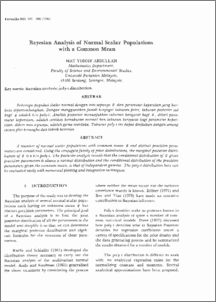# Bayesian Analysis of Normal Scalar Populations with a Common Mean

## Citation

Abdullah, Mat Yusoff (1986) Bayesian Analysis of Normal Scalar Populations with a Common Mean. Pertanika, 9 (3). pp. 381-386.

## Abstract

A number of normal scalar populations with common mean () and distinct precision parameters are considered. Using the conjugate family of prior distributions, the marginal posterior distribution of (0) is a k/o poly-to The posterior analysis reveals that the conditional distribution of (0) given precision parameters lS always a normal distribution and the conditional distribution of the precision parameters given the common mean, is that of independent gamma. The poly-t distribution here can be evaluated easily with numerical plotting and integration techniques.Preview
PDF
Bayesian_Analysis_of_Normal_Scalar_Populations.pdfView Item